# CTF | 2020 Ha1cyon_CTF公开赛 WriteUp

## 前言

（后面再看心情会不会按照大佬们的 WP 复现一下

## Misc

### HappyCheckInVerification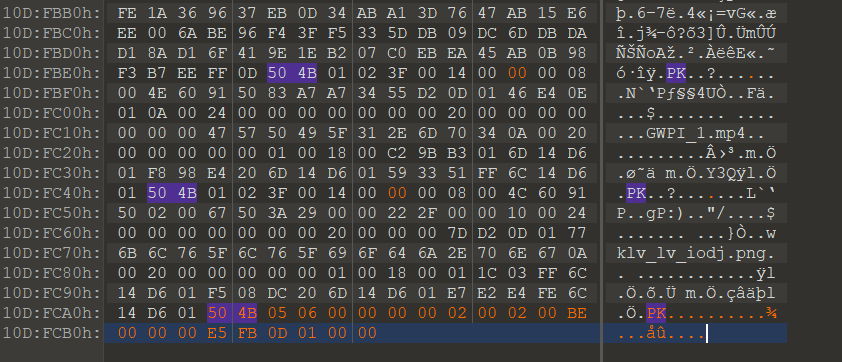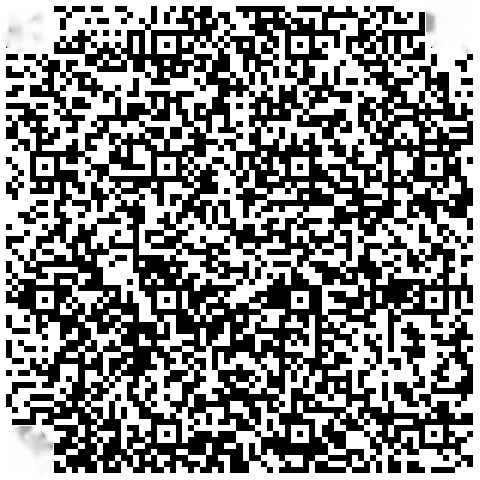``?flag{this_is_not_flag}三曳所諳陀怯耶南夜缽得醯怯勝數不知喝盧瑟侄盡遠故隸怯薩不娑羯涅冥伊盧耶諳提度奢道盧冥以朋罰所即栗諳蒙集皤夷夜集諳利顛呐寫無怯依奢竟#￥#%E68BBFE4BD9BE68B89E6A0BCE79A84E5A7BFE58ABFE59CA8E69C80E5908E32333333||254333254242254338254342254231254338254345254432254238254643254236254145254239254441254437254234254232254131254236254245253244253244254343254438254330254341254336254435...sadwq#asdsadasf faf\$use\$dasdasdafafa_\$ba##se64\$``

``三曳所諳陀怯耶南夜缽得醯怯勝數不知喝盧瑟侄盡遠故隸怯薩不娑羯涅冥伊盧耶諳提度奢道盧冥以朋罰所即栗諳蒙集皤夷夜集諳利顛呐寫無怯依奢竟``

``E68BBFE4BD9BE68B89E6A0BCE79A84E5A7BFE58ABFE59CA8E69C80E5908E32333333``

``254333254242254338254342254231254338254345254432254238254643254236254145254239254441254437254234254232254131254236254245253244253244254343254438254330254341254336254435``

base16 得到：

%C3%BB%C8%CB%B1%C8%CE%D2%B8%FC
%B6%AE%B9%DA%D7%B4%B2%A1%B6%BE
%2D%2D%CC%D8%C0%CA%C6%D5

（这里气死我了，居然用的 gb2312 编码而不是 UTF-8，我以为假的半天解不出来，虽然的确没啥用…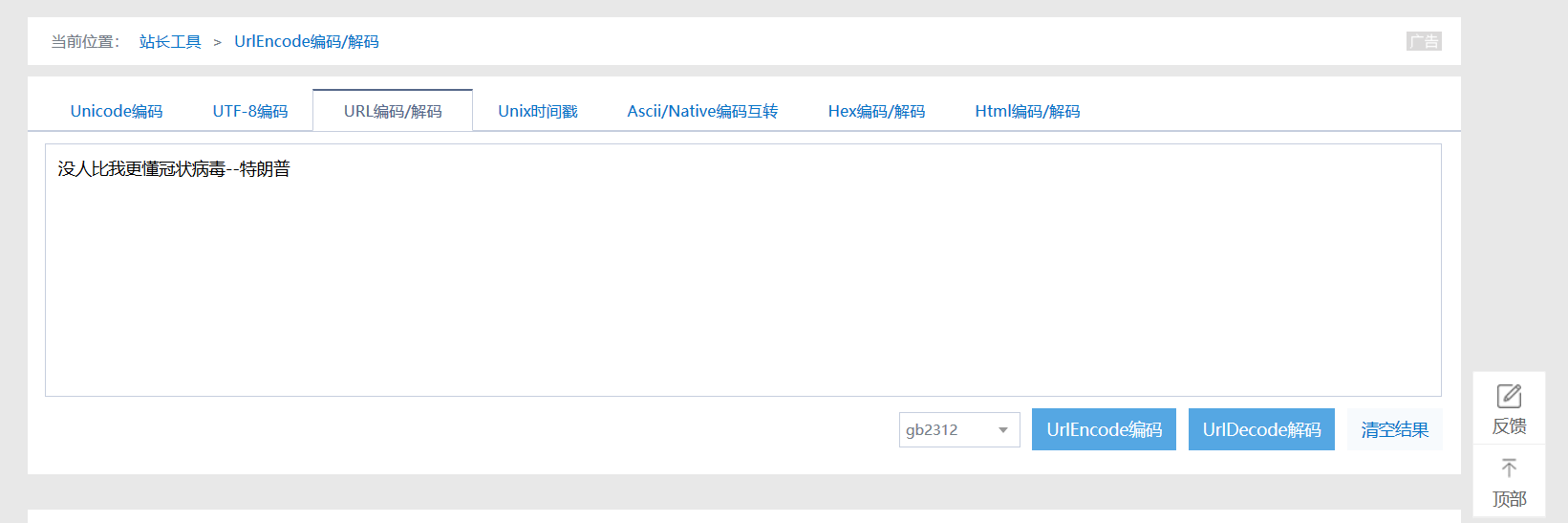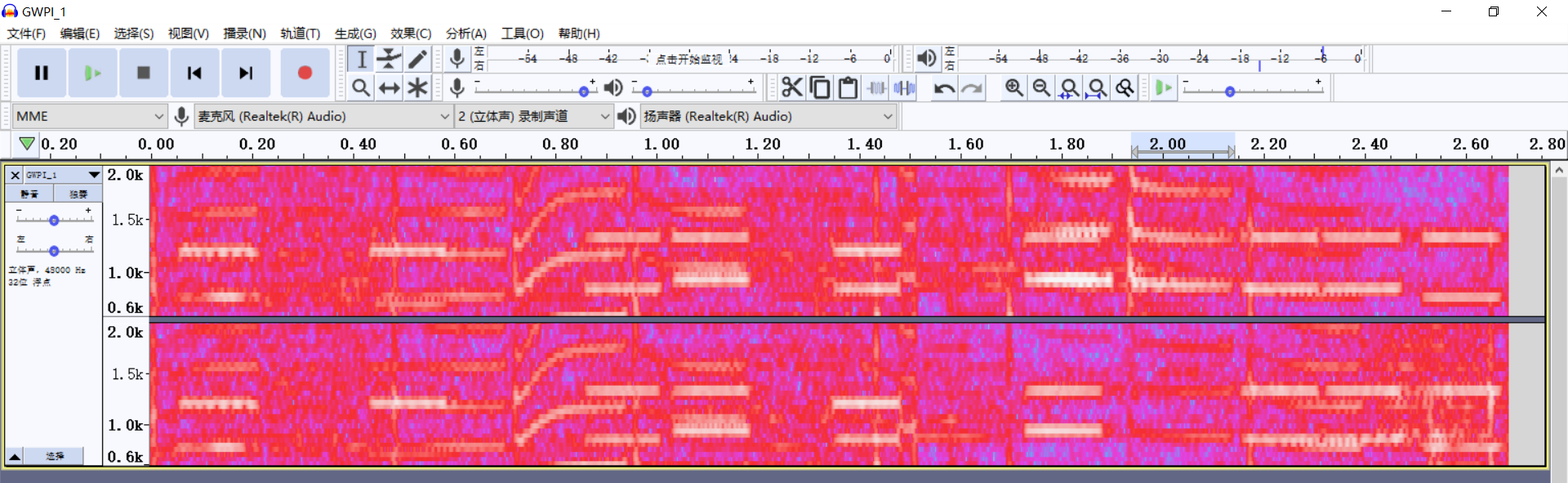1209 Hz 1336 Hz 1477 Hz 1633 Hz
697 Hz 1 2 3 A
770 Hz 4 5 6 B
852 Hz 7 8 9 C
941 Hz * 0 # D

（是 `13418070885`

base64 -> `NDE4MDcwODg1` `MTM0MTgwNzA4ODU=`

By the way，抛开题目来说，这个 BGM 就属于电音嘛，就一堆有规律的频率信号的组合唉，还带有连续调频。。

（真想不出来，啊是无线电大佬！Misc 真的杂。### 抽象带师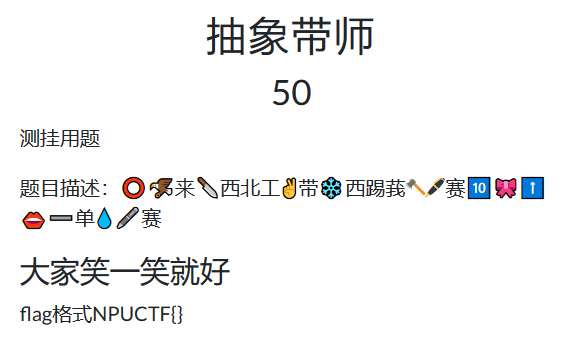`NPUCTF{欢迎来到西北工业大学CTF比赛世界上最简单的比赛}`

### parts

``````Here's the hint:
33 * 33 = 1089
And everything is inside the image``````foremost 提取不出来，看 Hex 文件是有点问题。

### 吃了它

`may be hint.txt`提示

``````emmmmm...
something important was hidden in secret, but I can't find it!``````

`lookatme.txt`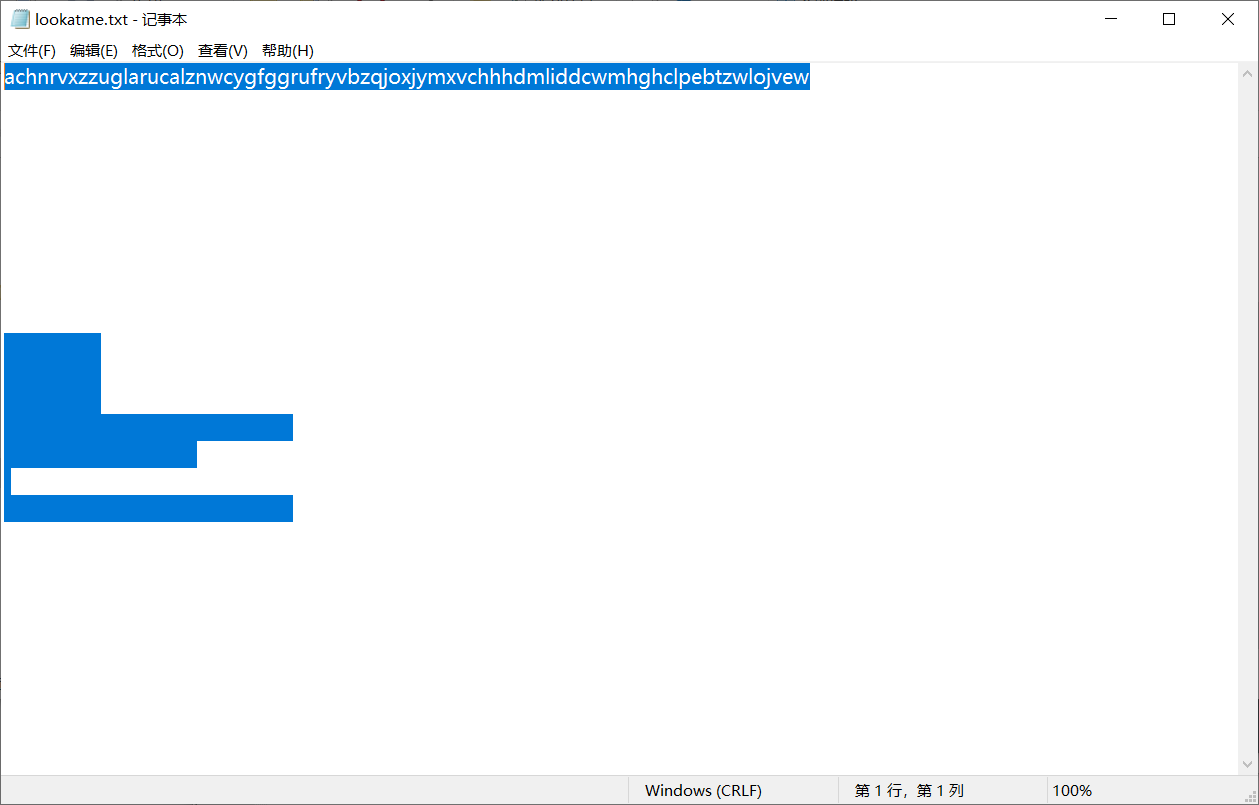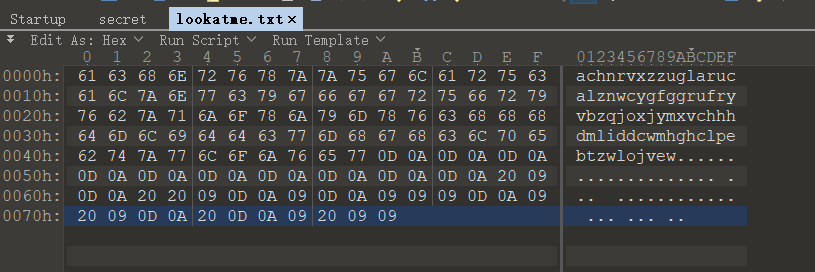`secret`文件头是 `MPE1`（4D 50 45 31），搜了一下没找到相关的文件格式呢。

## Web

### 查源码

F12 禁掉了，用 `Ctrl + Shift + C` 或者点浏览器的 Web 开发者 之类的就行。

## Crypto

### Classical_Cipher

`key.txt` 中提示：

``````解密后的flag请用flag{}包裹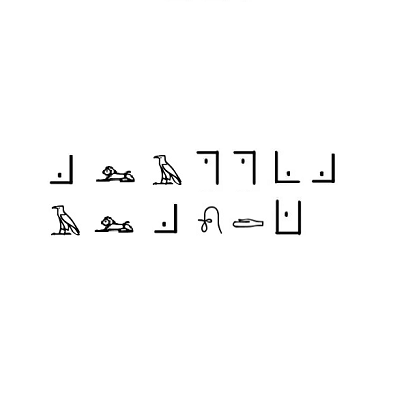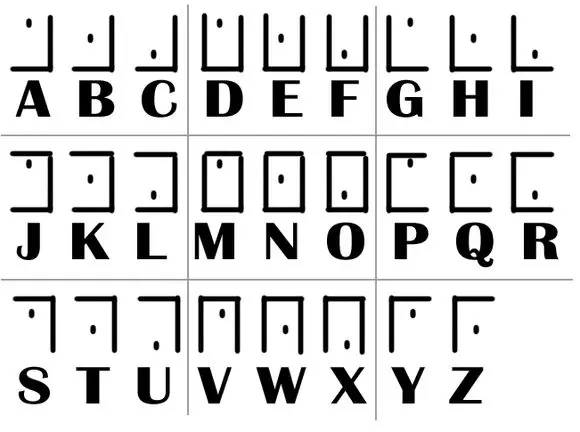`C??SSIC??C??E`Egypt0 Regular 里没找到，倒是在 ROSETTA STONE Regular 里找到了。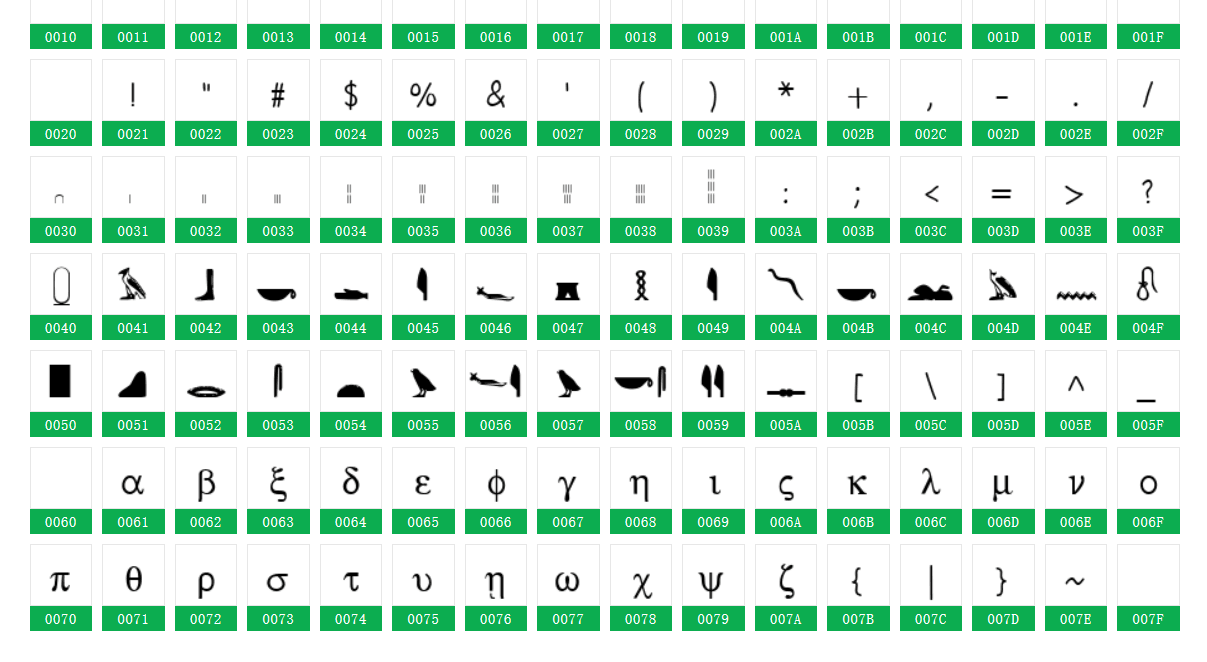`flag{classicalcode}`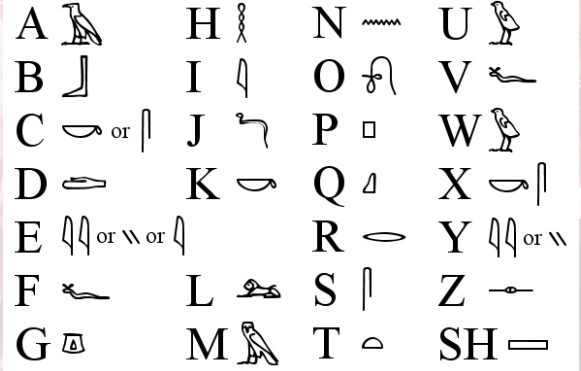### 这是什么觅🐎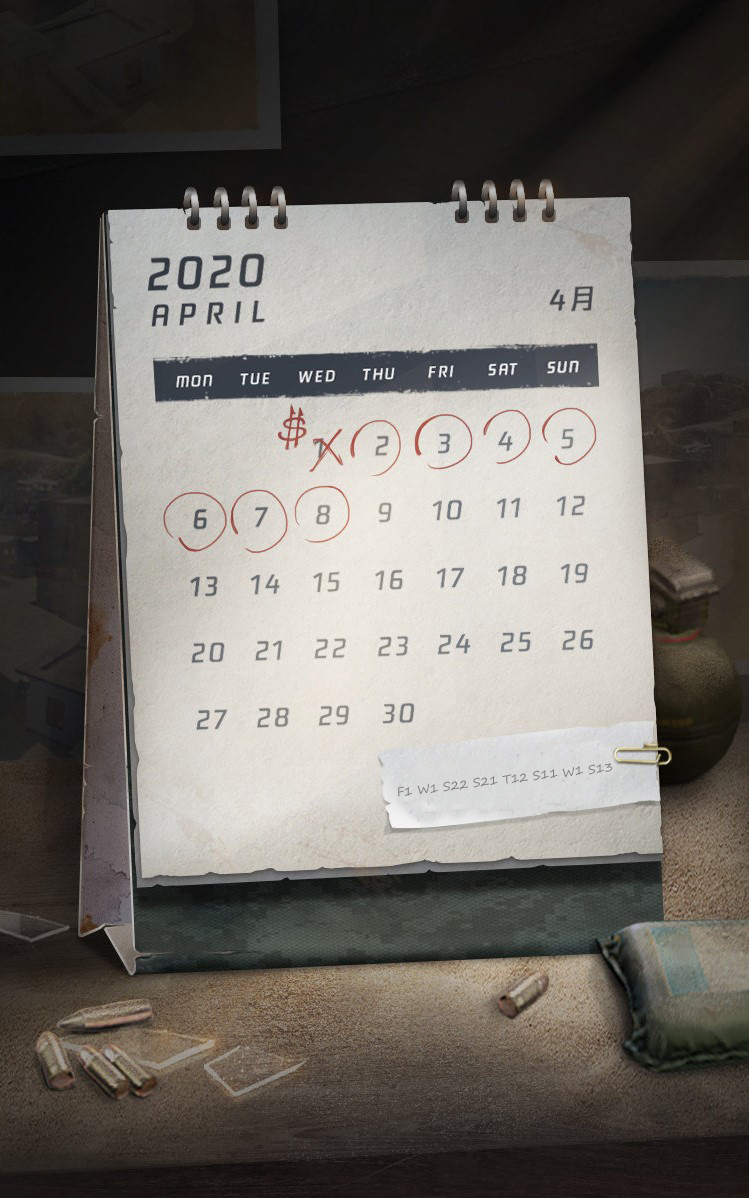2020年4月

F1 W1 S22 S21 T12 S11 W1 S13

3 1 12 5 14 4 1 18

``````l=[3,1,12,5,14,4,1,18]
s=''
for i in l:
s+=chr(ord('A')+i-1)
print(s)``````

`flag{calendar}`

（1）1—26代表a—z26个英文字母；（2）M,T1,W,T2,F,S1,S2分别代表周一到周日七天（英文首字母代替，1，2为了有别，也人为增加密度）；（3）密钥即密码表

4 9 3 20 9 15 14 1 18 25

S21 F2 S11 T13 F2 T23 W2 T21 S23 S24

（1）首先要知道密钥，即密码表；
（2）其次分解密码中的星期 i，即变成星期+数字的形式；
（3）去寻找对应的是第几个出现的星期 i，对应日期；
（4）对应字母，练成单词，短语或句子。

### 认清形势，建立信心

``````from Crypto.Util.number import *
from gmpy2 import *
from secret import flag

p = getPrime(25)
e = # Hidden
q = getPrime(25)
n = p * q
m = bytes_to_long(flag.strip(b"npuctf{").strip(b"}"))

c = pow(m, e, n)
print(c)
print(pow(2, e, n))
print(pow(4, e, n))
print(pow(8, e, n))

'''
169169912654178
128509160179202
518818742414340
358553002064450
'''``````

`Crypto`模块都不大熟有哪些现成方法，`gmpy2` 模块装了半天还没装好。。

## Reverse

### machine（不记得题目了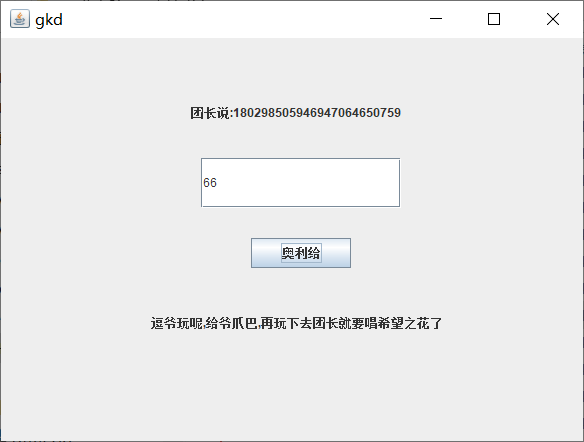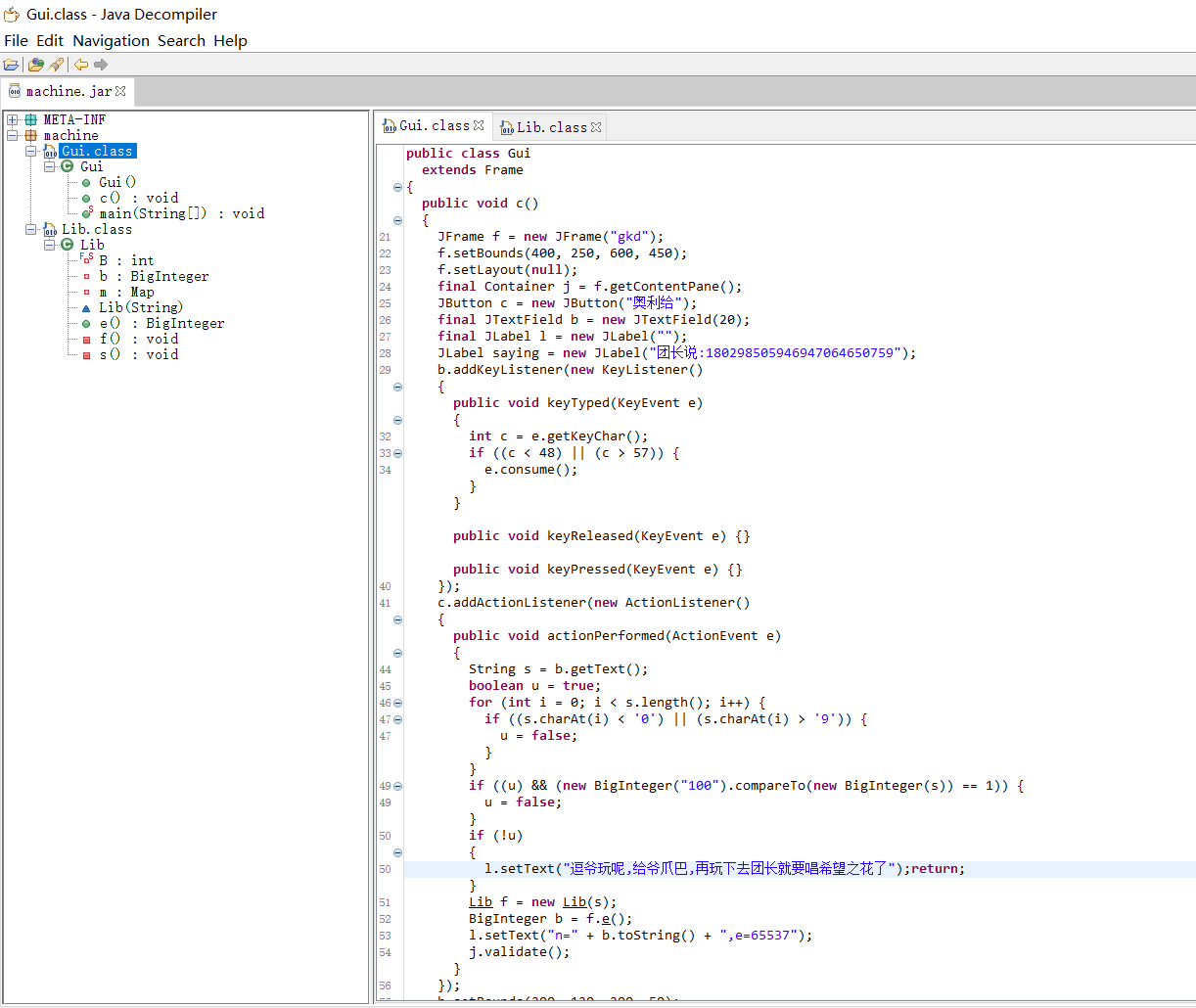``````public class Gui
extends Frame
{
public void c()
{
JFrame f = new JFrame("gkd");
f.setBounds(400, 250, 600, 450);
f.setLayout(null);
final Container j = f.getContentPane();
JButton c = new JButton("奥里给");
final JTextField b = new JTextField(20);
final JLabel l = new JLabel("");
JLabel saying = new JLabel("团长说:180298505946947064650759");
b.addKeyListener(new KeyListener()
{
public void keyTyped(KeyEvent e)
{
int c = e.getKeyChar();
if ((c < 48) || (c > 57)) {
e.consume();
}
}

public void keyReleased(KeyEvent e) {}

public void keyPressed(KeyEvent e) {}
});
c.addActionListener(new ActionListener()
{
public void actionPerformed(ActionEvent e)
{
String s = b.getText();
boolean u = true;
for (int i = 0; i < s.length(); i++) {
if ((s.charAt(i) < '0') || (s.charAt(i) > '9')) {
u = false;
}
}
if ((u) && (new BigInteger("100").compareTo(new BigInteger(s)) == 1)) {
u = false;
}
if (!u)
{
l.setText("逗爷玩呢,给爷爪巴,再玩下去团长就要唱希望之花了");return;
}
Lib f = new Lib(s);
BigInteger b = f.e();
l.setText("n=" + b.toString() + ",e=65537");
j.validate();
}
});
b.setBounds(200, 120, 200, 50);
c.setBounds(250, 200, 100, 30);
l.setBounds(150, 260, 300, 50);
saying.setBounds(190, 60, 220, 30);
j.add(b);
j.add(c);
j.add(l);
j.add(saying);
f.setDefaultCloseOperation(3);
f.setVisible(true);
}``````

``````Lib f = new Lib(s);
BigInteger b = f.e();
l.setText("n=" + b.toString() + ",e=65537");``````

``````package machine;

import java.math.BigInteger;
import java.util.HashMap;
import java.util.Map;
import java.util.Random;
import java.util.Set;
import java.util.Stack;

public class Lib
{
private BigInteger b;
private Map<BigInteger, Integer> m;
private static final int B = 150;

private void f()
{
BigInteger T = new BigInteger("3");
BigInteger t = new BigInteger("2");
BigInteger t1 = new BigInteger("0");
for (int i = 0; this.b.mod(t).equals(t1); i++) {
this.b = this.b.divide(t);
}
this.m.put(t, Integer.valueOf(i));
for (i = 0; this.b.mod(T).equals(t1); i++) {
this.b = this.b.divide(T);
}
this.m.put(T, Integer.valueOf(i));
}

private void s()
{
while (!this.b.equals(BigInteger.ONE))
{
BigInteger d = new BigInteger(150, new Random());
for (int i = 0; i < 3; i++) {
d = d.add(BigInteger.ONE);
}
if (this.b.mod(d).equals(BigInteger.ZERO))
{
this.b = this.b.divide(d);
for (BigInteger j = new BigInteger("5"); d.compareTo(j) == 1; j.add(BigInteger.ONE)) {
if (d.mod(j).equals(BigInteger.ZERO))
{
this.m.replace(j, Integer.valueOf(((Integer)this.m.get(j)).intValue() + 1));
j = j.subtract(BigInteger.ONE);
d = d.divide(j);
}
}
this.m.replace(d, Integer.valueOf(((Integer)this.m.get(d)).intValue() + 1));
}
}
}

public BigInteger e()
{
Set<BigInteger> p = this.m.keySet();
Stack<BigInteger> o = new Stack();
BigInteger d = BigInteger.ONE;
for (BigInteger q : p) {
o.push(q);
}
d = d.multiply((BigInteger)o.elementAt(o.size() - 1));
d = d.multiply((BigInteger)o.elementAt(o.size() - 2));
return d;
}

Lib(String num)
{
this.b = new BigInteger(num);
this.m = new HashMap();
f();
s();
}
}``````

`f()`函数先是不断让 b/2 直到 b mod 2 == 0，然后把这时的 2 和索引 i 放入 m；之后同理让 b/3，把 3 和索引 i 放入 m。

`s()`函数……

（做到这里后面有事就没看下去了

## 其他题

Reverse 里有个 `R_E_5_E_T.exe` 是用 VB 写的，神器 IDA 就直接用不了了，貌似还加了壳，给了一堆 hint 然而并不会做。

Crypto 里有个 `EzRSA`，文件名是 `difficultrsa.py`，噢暴露真相了2333。

## 小结

Misc 题一堆密码题，哇呜不会做……

A Python Interpreter Written in Python

Web 题用 nodejs 伪造 PHP 可还行，然而真好，都是我不会的。

（溜了溜了

上一篇CTF | 2020 DASCTF 四月春季战 Re&Misc WriteUp

2020-04-25数据科学 | 十分钟入门pandas/常用方法笔记

2020-04-17
目录